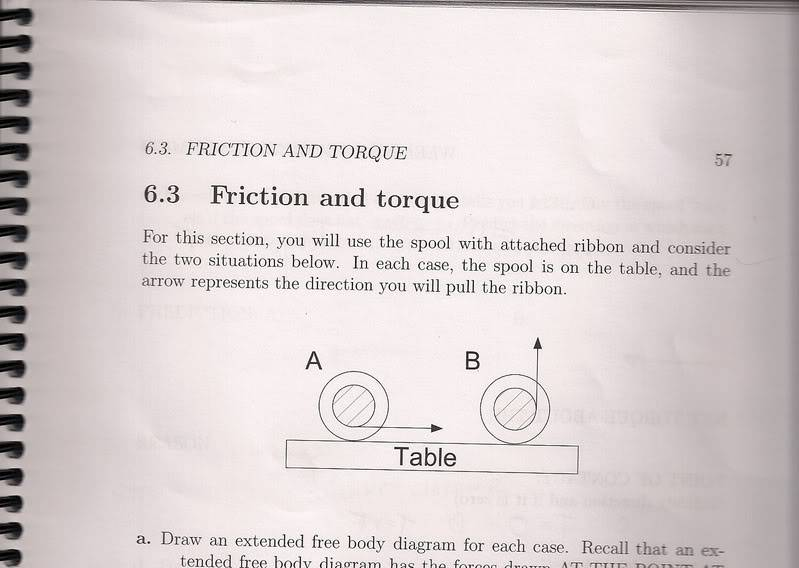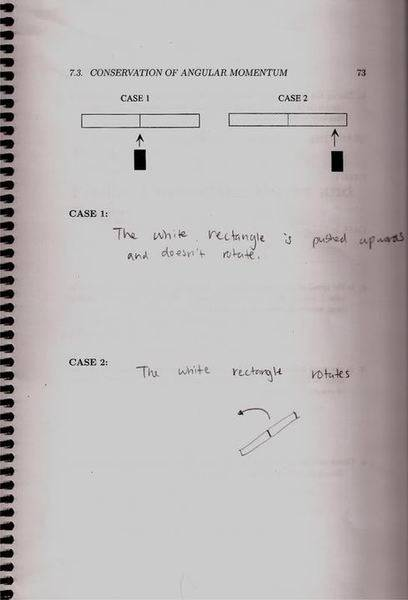# A couple basic conceptual questions (1 Viewer)

### Users Who Are Viewing This Thread (Users: 0, Guests: 1)

#### haydn

Question 1:I was told here that in Case A, the torque is equal to zero. I'm not really seeing that. I know torque is equal to rFsin$$\theta$$. If I draw a vector from the rotation point (the very center of the spool) to the point where the force is being applied, I get that the force and r are perpendicular, so sin$$\theta$$=1. Where am I going wrong? I was told that the torque in case B is rF, which I can clearly see by the same reasoning.

Question 2:For both cases, I was told the net force and net torque on the system is 0. The system is considered to be both of the objects. If someone could explain this I'd appreciate it.

Thanks.

#### tiny-tim

Homework Helper
"net"

I was told here that in Case A, the torque is equal to zero. I'm not really seeing that.

For both cases, I was told the net force and net torque on the system is 0. The system is considered to be both of the objects. If someone could explain this I'd appreciate it.
Hi haydn!(i'm assuming that the friction is preventing the spool from moving)

If the spool isn't moving, then yes the net torque is zero.

The net torque is the sum of the torque from the ribbon and the torque (or moment … same thing) from the friction force (and, technically the torque of the weight also, though if you take torque about the centre, then that will be zero)

Question 2 is similar … you use the net force or torque, which means adding all the forces or torques.#### haydn

Thanks for the reply. I'm not sure I'm understanding your explanation completely. Please tell me if I'm right or wrong here.

For my first question, in case A, the net torque is 0 because the friction force and the force applied via the ribbon cancel each other out. So incorporating the equation t=rFsin$$\theta$$, you would plug the net force, 0 into the equation?

Then for case B on the second question though, there are two force components, friction and the force applied via the ribbon... wouldn't this result in a net force at some angle other than 90 degrees? I was told thought that for case B, torque is just equal to rF.

Thanks for the help.

#### tiny-tim

Homework Helper
I'm really not following what you're doing.

The question quite clearly tells you to start by drawing "an extended free body diagram for each case".

Have you done this? What does it show? What equations can you get from it?

#### haydn

Yes I drew free body diagrams. For case A I have a force going to the right via the ribbon and a friction force going to the left. I believe these cancel out for a net force of 0.

For case B I have a force going straight up via the ribbon and a friction force perpendicular to that, going to the left along the table. I don't get how the net torque in this case is equal to rF.

#### tiny-tim

Homework Helper
Yes I drew free body diagrams.
No you didn't …

what about the other forces?​

### The Physics Forums Way

We Value Quality
• Topics based on mainstream science
• Proper English grammar and spelling
We Value Civility
• Positive and compassionate attitudes
• Patience while debating
We Value Productivity
• Disciplined to remain on-topic
• Recognition of own weaknesses
• Solo and co-op problem solving